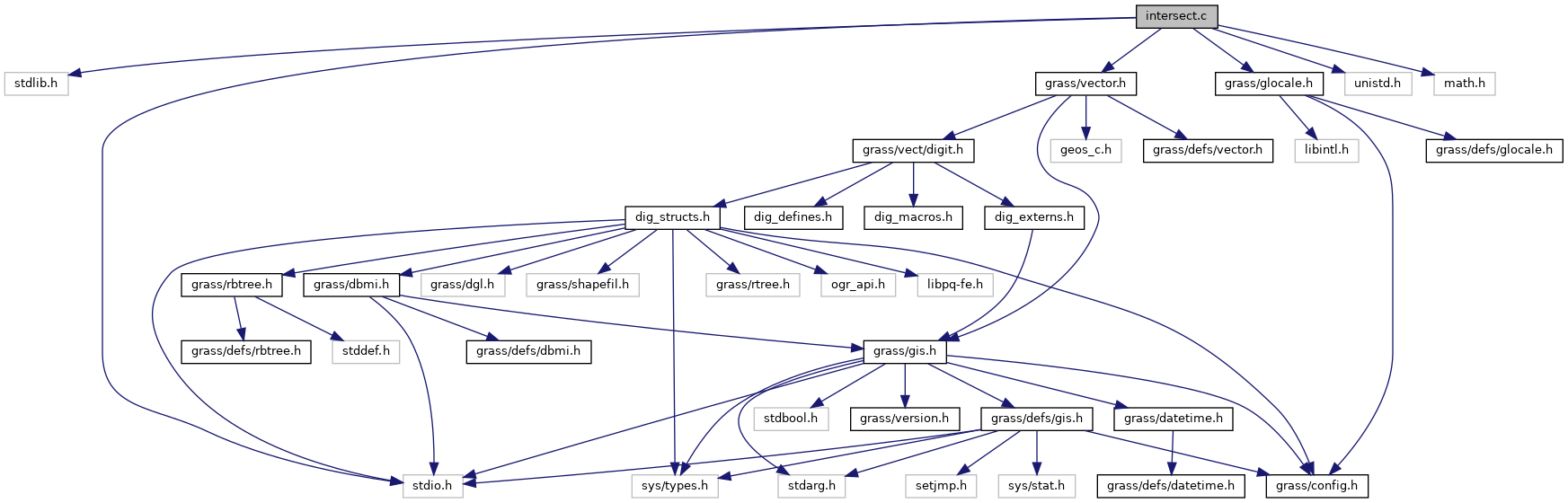GRASS GIS 7 Programmer's Manual  7.9.dev(2021)-e5379bbd7
vector/Vlib/intersect.c File Reference

Vector library - intersection. More...

`#include <stdlib.h>`
`#include <stdio.h>`
`#include <unistd.h>`
`#include <math.h>`
`#include <grass/vector.h>`
`#include <grass/glocale.h>`
Include dependency graph for vector/Vlib/intersect.c:Go to the source code of this file.

## Macros

#define D   ((ax2-ax1)*(by1-by2) - (ay2-ay1)*(bx1-bx2))

#define D1   ((bx1-ax1)*(by1-by2) - (by1-ay1)*(bx1-bx2))

#define D2   ((ax2-ax1)*(by1-ay1) - (ay2-ay1)*(bx1-ax1))

## Functions

int Vect_segment_intersection (double ax1, double ay1, double az1, double ax2, double ay2, double az2, double bx1, double by1, double bz1, double bx2, double by2, double bz2, double *x1, double *y1, double *z1, double *x2, double *y2, double *z2, int with_z)
Check for intersect of 2 line segments. More...

int Vect_line_intersection (struct line_pnts *APoints, struct line_pnts *BPoints, struct bound_box *ABox, struct bound_box *BBox, struct line_pnts ***ALines, struct line_pnts ***BLines, int *nalines, int *nblines, int with_z)
Intersect 2 lines. More...

int Vect_line_check_intersection (struct line_pnts *APoints, struct line_pnts *BPoints, int with_z)
Check if 2 lines intersect. More...

int Vect_line_get_intersections (struct line_pnts *APoints, struct line_pnts *BPoints, struct line_pnts *IPoints, int with_z)
Get 2 lines intersection points. More...

## Detailed Description

Vector library - intersection.

Higher level functions for reading/writing/manipulating vectors.

Some parts of code taken from grass50 v.spag/linecros.c

Based on the following:

` (ax2-ax1)r1 - (bx2-bx1)r2 = ax2 - ax1 (ay2-ay1)r1 - (by2-by1)r2 = ay2 - ay1 `

Solving for r1 and r2, if r1 and r2 are between 0 and 1, then line segments (ax1,ay1)(ax2,ay2) and (bx1,by1)(bx2,by2) intersect.

Intersect 2 line segments.

Returns: 0 - do not intersect 1 - intersect at one point

```\  /    \  /  \  /
\/      \/    \/
/\             \
/  \             \
2 - partial overlap         ( \/                      )
------      a          (    distance < threshold )
------   b          (                         )
3 - a contains b            ( /\                      )
----------  a    ----------- a
----     b          ----- b
4 - b contains a
----     a          ----- a
----------  b    ----------- b
5 - identical
----------  a
----------  b
```

Intersection points:

```return  point1 breakes: point2 breaks:    distance1 on:   distance2 on:
0        -              -                  -              -
1        a,b            -                  a              b
2        a              b                  a              b
3        a              a                  a              a
4        b              b                  b              b
5        -              -                  -              -
```

Sometimes (often) is important to get the same coordinates for a x b and b x a. To reach this, the segments a,b are 'sorted' at the beginning, so that for the same switched segments, results are identical. (reason is that double values are always rounded because of limited number of decimal places and for different order of coordinates, the results would be different)

(C) 2001-2009 by the GRASS Development Team

This program is free software under the GNU General Public License (>=v2). Read the file COPYING that comes with GRASS for details.

Definition in file vector/Vlib/intersect.c.

## ◆ D

 #define D   ((ax2-ax1)*(by1-by2) - (ay2-ay1)*(bx1-bx2))

Definition at line 89 of file vector/Vlib/intersect.c.

## ◆ D1

 #define D1   ((bx1-ax1)*(by1-by2) - (by1-ay1)*(bx1-bx2))

Definition at line 90 of file vector/Vlib/intersect.c.

## ◆ D2

 #define D2   ((ax2-ax1)*(by1-ay1) - (ay2-ay1)*(bx1-ax1))

Definition at line 91 of file vector/Vlib/intersect.c.

## ◆ Vect_line_check_intersection()

 int Vect_line_check_intersection ( struct line_pnts * APoints, struct line_pnts * BPoints, int with_z )

Check if 2 lines intersect.

Points (Points->n_points == 1) are also supported.

Parameters
 APoints first input line BPoints second input line with_z 3D, not supported (only if one or both are points)!
Returns
0 no intersection
1 intersection found

Definition at line 1275 of file vector/Vlib/intersect.c.

Referenced by Vect_line_get_intersections().

## ◆ Vect_line_get_intersections()

 int Vect_line_get_intersections ( struct line_pnts * APoints, struct line_pnts * BPoints, struct line_pnts * IPoints, int with_z )

Get 2 lines intersection points.

A wrapper around Vect_line_check_intersection() function.

Parameters
 APoints first input line BPoints second input line [out] IPoints output with intersection points with_z 3D, not supported (only if one or both are points)!
Returns
0 no intersection
1 intersection found

Definition at line 1458 of file vector/Vlib/intersect.c.

References Vect_line_check_intersection().

## ◆ Vect_line_intersection()

 int Vect_line_intersection ( struct line_pnts * APoints, struct line_pnts * BPoints, struct bound_box * ABox, struct bound_box * BBox, struct line_pnts *** ALines, struct line_pnts *** BLines, int * nalines, int * nblines, int with_z )

Intersect 2 lines.

Creates array of new lines created from original A line, by intersection with B line. Points (Points->n_points == 1) are not supported.

Parameters
 APoints first input line BPoints second input line [out] ALines array of new lines created from original A line [out] BLines array of new lines created from original B line [out] nalines number of new lines (ALines) [out] nblines number of new lines (BLines) with_z 3D, not supported!
Returns
0 no intersection
1 intersection found

Definition at line 650 of file vector/Vlib/intersect.c.

References l, x, and line_pnts::y.

## ◆ Vect_segment_intersection()

 int Vect_segment_intersection ( double ax1, double ay1, double az1, double ax2, double ay2, double az2, double bx1, double by1, double bz1, double bx2, double by2, double bz2, double * x1, double * y1, double * z1, double * x2, double * y2, double * z2, int with_z )

Check for intersect of 2 line segments.

Parameters
 ax1,ay1,az1,ax2,ay2,az2 input line a bx1,by1,bz1,bx2,by2,bz2 input line b [out] x1,y1,z1 intersection point1 (case 2-4) [out] x2,y2,z2 intersection point2 (case 2-4) with_z use z coordinate (3D) (TODO)
Returns
0 - do not intersect,
1 - intersect at one point,
2 - partial overlap,
3 - a contains b,
4 - b contains a,
5 - identical

Definition at line 109 of file vector/Vlib/intersect.c.